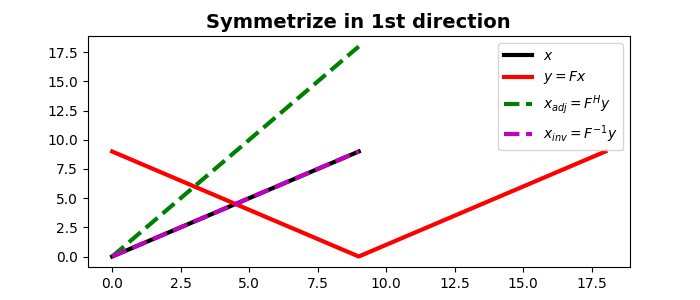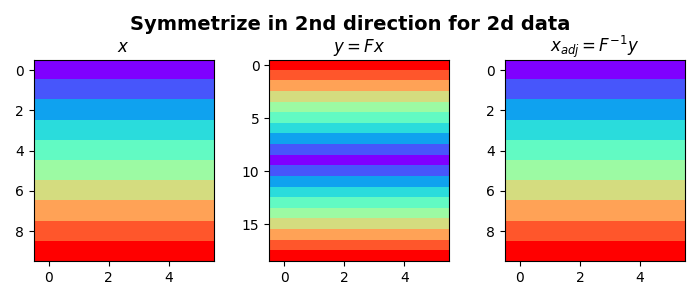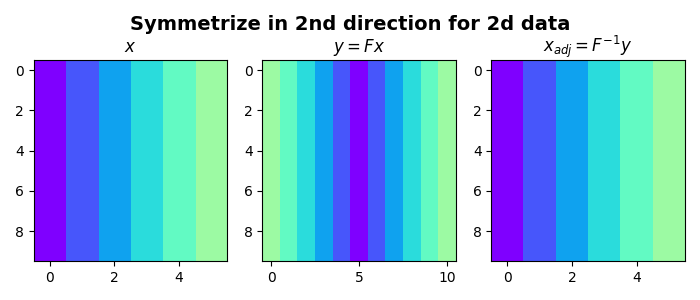# Symmetrize¶

This example shows how to use the pylops.Symmetrize operator which takes an input signal and returns a symmetric signal by pre-pending the input signal in reversed order. Such an operation can be inverted as we will see in this example.

Moreover the pylops.Symmetrize can be used as preconditioning to any inverse problem where we are after inverting for a signal that we want to ensure is symmetric. Refer to Wavelet estimation for an example of such a type.

import matplotlib.pyplot as plt
import numpy as np

import pylops

plt.close("all")


Let’s start with a 1D example. Define an input signal composed of nt samples

nt = 10
x = np.arange(nt)


We can now create our flip operator and apply it to the input signal. We can also apply the adjoint to the flipped signal and we can see how for this operator the adjoint is effectively equivalent to the inverse.

Sop = pylops.Symmetrize(nt)
y = Sop * x
xadj = Sop.H * y
xinv = Sop / y

plt.figure(figsize=(7, 3))
plt.plot(x, "k", lw=3, label=r"$x$")
plt.plot(y, "r", lw=3, label=r"$y=Fx$")
plt.plot(xadj, "--g", lw=3, label=r"$x_{adj} = F^H y$")
plt.plot(xinv, "--m", lw=3, label=r"$x_{inv} = F^{-1} y$")
plt.title("Symmetrize in 1st direction", fontsize=14, fontweight="bold")
plt.legend()Out:

<matplotlib.legend.Legend object at 0x7f4dec9be668>


Let’s now repeat the same exercise on a two dimensional signal. We will first flip the model along the first axis and then along the second axis

nt, nx = 10, 6
x = np.outer(np.arange(nt), np.ones(nx))

Sop = pylops.Symmetrize(nt * nx, dims=(nt, nx), dir=0)
y = Sop * x.ravel()
xadj = Sop.H * y.ravel()
xinv = Sop / y
y = y.reshape(2 * nt - 1, nx)
xinv = xinv.reshape(nt, nx)

fig, axs = plt.subplots(1, 3, figsize=(7, 3))
fig.suptitle(
"Symmetrize in 2nd direction for 2d data", fontsize=14, fontweight="bold", y=0.95
)
axs.imshow(x, cmap="rainbow", vmin=0, vmax=9)
axs.set_title(r"$x$")
axs.axis("tight")
axs.imshow(y, cmap="rainbow", vmin=0, vmax=9)
axs.set_title(r"$y=Fx$")
axs.axis("tight")
axs.imshow(xinv, cmap="rainbow", vmin=0, vmax=9)
axs.set_title(r"$x_{adj}=F^{-1}y$")
axs.axis("tight")
plt.tight_layout()

x = np.outer(np.ones(nt), np.arange(nx))
Sop = pylops.Symmetrize(nt * nx, dims=(nt, nx), dir=1)

y = Sop * x.ravel()
xadj = Sop.H * y.ravel()
xinv = Sop / y
y = y.reshape(nt, 2 * nx - 1)
xinv = xinv.reshape(nt, nx)

# sphinx_gallery_thumbnail_number = 3
fig, axs = plt.subplots(1, 3, figsize=(7, 3))
fig.suptitle(
"Symmetrize in 2nd direction for 2d data", fontsize=14, fontweight="bold", y=0.95
)
axs.imshow(x, cmap="rainbow", vmin=0, vmax=9)
axs.set_title(r"$x$")
axs.axis("tight")
axs.imshow(y, cmap="rainbow", vmin=0, vmax=9)
axs.set_title(r"$y=Fx$")
axs.axis("tight")
axs.imshow(xinv, cmap="rainbow", vmin=0, vmax=9)
axs.set_title(r"$x_{adj}=F^{-1}y$")
axs.axis("tight")
plt.tight_layout()

••